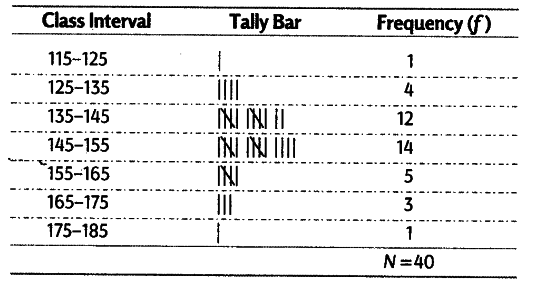# From the following data relating to weights of 40 students in kg

From the following data relating to weights of 40 students in kg
138,164,150,131,144,125,149,157,146,148,140,147,136,148,152,144,168,126,138,176, 163,119,150,165,146,173,142,147,135,153,142,135,140,135,161,145,150,156,145,128.
(i) Obtain the range of weights of the students.
(ii) Divide the range into appropriate number of class interval and obtain the frequency distribution.
(iii) Find the number of students whose weight is
(a) less than 145 kg (b) more than 155 kg © between 135 and 155 kg

(i) Range = 176-119 = 57
(ii) The frequency distribution by dividing into appropriate range is shown below(iii) Number of students whose weight is
(a) less than 145 kg = 17 (b) more than 155 kg = 9 © between 135 and 155 kg = 26